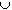#Interactive Real Analysis

Next | Previous | Glossary | Map

## 5.1. Open and Closed Sets

### Proposition 5.1.4: Characterizing Open Sets

Let UR be an arbitrary open set. Then there are countably many pairwise disjoint open intervals U n such that U =U n

### Proof:

This proposition is rather interesting, giving a complete description of any possible open set in the real line. To prove it, we will make use of equivalence relations and classes again. First, let us define a relation on U:
• if a and b are in U, we say that a ~ b if the whole line segment between a and b is also contained in U.Is this relation indeed an equivalence relation ? Assuming that it is, we know immediately that U equals the union of the equivalence classes, and the equivalence classes are pairwise disjoint. Denote those equivalent classes by U n

Each U n is an interval: take any two points a and b in U n. Being in the same equivalence classes, a and b must be related. But then the whole line segment between a and b is contained in U n as well. Since a and b were arbitrary, U n is indeed an interval.

Each U n is open: take any xU n. Then xU, and since U is open, there exists an> 0 such that ( x -, x +) is contained in U. But clearly each point in that interval is related to x, hence this neighborhood is contained in U n, proving that U n is open.

There are only countably many U n: This seems the hard part. But, each U n must contain at least one different rational number.Why ? Since there are only countably many rational numbers, there can only be countably many of the U n's (since they are disjoint).Next | Previous | Glossary | Map